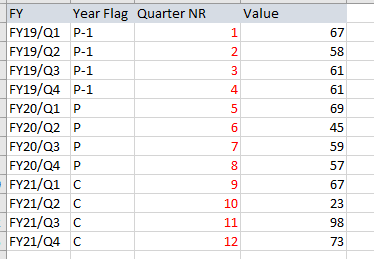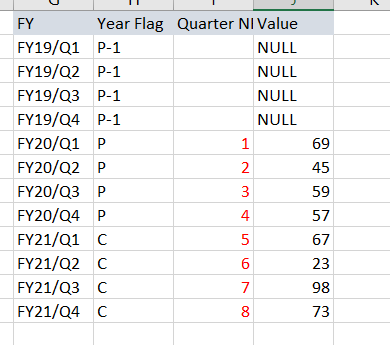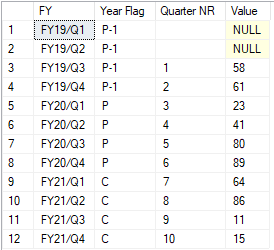# How to update a column in a different way based on 2 conditions

Hello,

i have a table in which I need to update the column [Quarter NR] but it depends on 2 conditions.1. if [Year Flag] is P-1 (the previous year of the past year) has the [value]<> NULL then the [Quarter NR] should be updated like in the first picture (I need to number the column starting 1 to 12 starting the [Year Flag]=P-1)
2. if the value is NULL for the P-1 then I should update the [Quarter NR] starting 1 to 8 (need to number the column starting 1 from the [Year Flag]=P)
it should look like this:
[FY] [Year Flag] [Quarter NR] [Value]
FY19/Q1 P-1 0 null
FY19/Q2 P-1 0 null
FY19/Q3 P-1 0 null
FY19/Q4 P-1 0 null
FY20/Q1 P 1 69
FY20/Q2 P 2 45
FY20/Q3 P 3 59
FY20/Q4 P 4 57
FY21/Q1 C 5 67
FY21/Q2 C 6 23
FY21/Q3 C 7 98
FY21/Q4 C 8 73

thank you in advanceDDL and sample data is so much easier to work with

DDL And Sample Data

Summary

create table #t (FY varchar(10),
YearFlag varchar(10),
QuarterNR int,
QValue int,
NewValue int,
NewQValue int)

insert into #t (FY, YearFlag, QuarterNR, QValue)
values
('FY19/Q1','P-1',1,67),
('FY19/Q2','P-1',2,58),
('FY19/Q3','P-1',3,61),
('FY19/Q4','P-1',4,61),
('FY20/Q1','P',5,69),
('FY20/Q2','P',6,45),
('FY20/Q3','P',7,59),
('FY20/Q4','P',8,57),
('FY21/Q1','C',9,67),
('FY21/Q2','C',10,23),
('FY21/Q3','C',11,98),
('FY21/Q4','C',12,73)

Update Statement

Summary

update t
set NewValue = Case when t.YearFlag = 'P-1' then null else t.QValue end,
NewQValue = Case when t.YearFlag = 'P-1' then 0 else RowNum end
from #T t
join (Select *, Row_number() over (partition by Case when YearFlag = 'P-1' then 1 else 0 end order by QuarterNR) as RowNUm
from #t) tt
on t.QuarterNR = tt.QuarterNR

Select * from #T

Hello,

here is a data sample:
create table Calendar
([FY]nvarchar(20) null,
[Year Flag]nvarchar(10)null,
[Quarter NR] int null,
[Value] float null)

insert into Calendar ([FY],[Year Flag],[Quarter NR],[Value])
values('FY19/Q1','P-1','','62')
( 'FY19/Q2','P-1','','67')
('FY19/Q3','P-1','','58')
('FY19/Q4','P-1','','61')
('FY20/Q1','P','','23')
('FY20/Q2','P','','41')
('FY20/Q3','P','','80')
('FY20/Q4','P','','89')
('FY21/Q1','C','','64')
('FY21/Q2','C','','86')
('FY21/Q3','C','','11')
('FY21/Q4','C','','15')

in this case the [Quarter NR] should be filled from 1 to 12 like it is in my first picture.
My problem is that I don't know how to switch if the values from P-1 are null like in this pic:I wrote this:
update [dbo].[Calendar ]
set [Quarter NR] = case when [Value]is not null and [Year Flag]='P-1' and [Quarter]='Q1' then '1'
when [Value]is not null and [Year Flag]='P-1' and [Quarter]='Q2' then '2'
when [Value]is not null and [Year Flag]='P-1' and [Quarter]='Q3' then '3'
when [Value]is not null and [Year Flag]='P-1' and [Quarter]='Q4' then '4'
when [Year Flag]='P' and [Quarter]='Q1' then '5'
when [Year Flag]='P' and [Quarter]='Q2' then '6'
when [Year Flag]='P' and [Quarter]='Q3' then '7'
when [Year Flag]='P' and [Quarter]='Q4' then '8'
when [Year Flag]='C' and [Quarter]='Q1' then '9'
when [Year Flag]='C' and [Quarter]='Q2' then '10'
when [Year Flag]='C' and [Quarter]='Q3' then '11'
when [Year Flag]='C' and [Quarter]='Q4' then '12'
end
my problem is that I don't know how to make the switch in case the values for P-1 is null

Your example does not have any rows with NULL values...this is what I assume you are looking for:

``````Declare @Calendar Table (
[FY] nvarchar(20) Null
, [Year Flag] nvarchar(10) Null
, [Quarter NR] int Null
, [Value] float Null);

Insert Into @Calendar ([FY], [Year Flag], [Quarter NR], [Value])
Values ('FY19/Q1', 'P-1', '', Null) -- '62')
, ('FY19/Q2', 'P-1', '', Null) --'67')
, ('FY19/Q3', 'P-1', '', Null) --'58')
, ('FY19/Q4', 'P-1', '', Null) --'61')
, ('FY20/Q1', 'P', '', '23')
, ('FY20/Q2', 'P', '', '41')
, ('FY20/Q3', 'P', '', '80')
, ('FY20/Q4', 'P', '', '89')
, ('FY21/Q1', 'C', '', '64')
, ('FY21/Q2', 'C', '', '86')
, ('FY21/Q3', 'C', '', '11')
, ('FY21/Q4', 'C', '', '15');

With sumP1Values
As (
Select Total = sum(c.[Value])
From @Calendar c
Where [Year Flag] = 'P-1'
)
Select c.FY
, c.[Year Flag]
, [Quarter NR] = Case When c.[Year Flag] = 'P-1' And sp.Total Is Null Then ''
Else cast(row_number() over(Partition By iif(c.[Year Flag] = 'P-1' And sp.Total Is Null, 0, 1) Order By c.[FY]) As varchar(2))
End
, c.[Value]
From @Calendar c
Cross Join sumP1Values sp
``````

hello
the [Value] column is calculated based on the [FY]column. in my example i put values for all the fiscal years. My table is being updated automatically based on the refresh date. So, based on the day in which i make the refresh, the [FY] column will be updated automatically, also the [Year Flag]. The Value is updated by taking the data from another table with a left join, having as key the [FY] column. So sometimes i will have data in the [Year Flag]=P-1 and sometimes i wont have data for P-1, it will be Null. If i will have data , then i must start to update the [Quarter NR] from P-1, and if i have NULL for P-1 then i must update the [Quarter NR] starting [Year Flag]=P.
Hope that now am am more clearIf you have data for P-1, will you always have data for all quarters? If not - do you want to start counting at the first non-null quarter or do you want to count for all quarters?

Are you only ever calculating the values for P-1 (Prior), P (Previous) and C (Current)? In other words - only those 12 quarters will be calculated when this code runs?

Here is another way to accomplish this:

`````` Select c.FY
, c.[Year Flag]
, [Quarter NR] = Case When c.[Value] Is Null Then ''
Else cast(row_number() over(Partition By iif(c.[Value] Is Null, 0, 1) Order By c.[FY]) As varchar(2))
End
, c.[Value]
From @Calendar                c;
``````

If you only ever calculate for those 3 years...

This version will start counting from the first non-null quarter...for example:

``````Declare @Calendar Table (
[FY] nvarchar(20) Null
, [Year Flag] nvarchar(10) Null
, [Quarter NR] int Null
, [Value] float Null);

Insert Into @Calendar ([FY], [Year Flag], [Quarter NR], [Value])
Values ('FY19/Q1', 'P-1', '', Null) -- '62')
, ('FY19/Q2', 'P-1', '', Null) --'67')
, ('FY19/Q3', 'P-1', '', 58) --'58')
, ('FY19/Q4', 'P-1', '', 61) --'61')
--Values ('FY19/Q1', 'P-1', '', '62')
--     , ('FY19/Q2', 'P-1', '', '67')
--     , ('FY19/Q3', 'P-1', '', '58')
--     , ('FY19/Q4', 'P-1', '', '61')
, ('FY20/Q1', 'P', '', '23')
, ('FY20/Q2', 'P', '', '41')
, ('FY20/Q3', 'P', '', '80')
, ('FY20/Q4', 'P', '', '89')
, ('FY21/Q1', 'C', '', '64')
, ('FY21/Q2', 'C', '', '86')
, ('FY21/Q3', 'C', '', '11')
, ('FY21/Q4', 'C', '', '15');

Select c.FY
, c.[Year Flag]
, [Quarter NR] = Case When c.[Value] Is Null Then ''
Else cast(row_number() over(Partition By iif(c.[Value] Is Null, 0, 1) Order By c.[FY]) As varchar(2))
End
, c.[Value]
From @Calendar                c;
``````

Results:thank you so much it works

thank you for the feedback